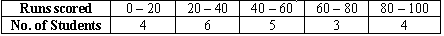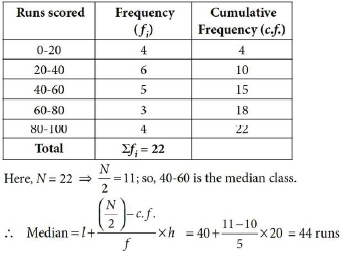# CBSE Class 10 Mathematics Term 2 Sample Paper 2022 Set B

Read and download PDF of CBSE Class 10 Mathematics Sample Paper Set W designed as per the latest curriculum and examination pattern for Class 10 issued by CBSE, NCERT and KVS. The latest Class 10 Mathematics Sample Papers have been provided with solutions so that the students can solve these practice papers and then compare their answers. This will help them to identify mistakes and improvement areas in Mathematics Standard 10 which they need to study more to get better marks in Grade 10 exams. After solving these guess papers also refer to solved Class 10 Mathematics Question Papers available on our website to build strong understanding of the subject

## Mathematics Sample Paper Class 10

Students can refer to the below Class 10 Mathematics Sample Paper designed to help students understand the pattern of questions that will be asked in Grade 10 exams. Please download CBSE Class 10 Mathematics Sample Paper Set W

### Mathematics Class 10 Sample Paper

SECTION – A

Question : Find the discriminant of the quadratic equation 3x2 − 2√2x − 2√3 = 0 .
OR
The sum of the squares of two consecutive natural numbers is 41. Represent this situation in the form of a quadratic equation.
Answer : The given equation is √3x2 − 2√2x − 2√3 = 0.
Here, a = √3, b = − 2√2 and c = − 2√3
Now, D = b2 – 4ac
D = (−2√2)2 − 4 × √3 × (−2√3) = 8 + 24 = 32
OR
The sum of the squares of two consecutive natural numbers is 41. Represent this situation in the form of a quadratic equation.
Answer :  Let the two consecutive natural numbers be x and x + 1.
Then, their squares are x2 and (x +1)2 respectively.
∴ The required equation is, x2 + (x + 1)2 = 41
⇒ x2 + x2 + 1 + 2x = 41
⇒ 2x2 + 2x – 40 = 0 ⇒ x2 + x – 20 = 0

Question : Find the roots of the quadratic equation x2 + 4√2x + 6 = 0.
Answer : We have, x2 + 4√2x + 6 = 0
⇒ x2 + 3√2 x + √2 x + 6 = 0
⇒ x(x + 3√2) + 2 (x + 3√2) = 0
⇒ (x + 2)(x + 3√2) = 0
⇒ x = − 2 or x = −3√2
∴ Roots are − 2 and − 3√2.

Question : XY and MN are the tangents drawn at the end points of the diameter DE of the circle with centre O. Prove that XY || MN.
Answer : Since, XY is the tangent to the circle at the point D.⇒ OD ⊥ XY ⇒ ∠EDX = 90°
Also, MN is the tangent to the circle at E.
⇒ OE ⊥ MN ⇒ ∠ DEN = 90°
As, ∠EDX = ∠DEN (each 90°)
which are alternate interior angles.
⇒ XY ||MN

Question : An inter house cricket match was organized by a school. Distribution of runs made by the students is given below. Find the median runs scored.Question : Find the common difference of the AP 4, 9, 14, … If the first term changes to 6 and the common difference remains the same then write the new AP.
Answer : Given A.P. is 4, 9, 14, ...
So, common difference, d = 9 – 4 = 5
If the first term is 6 and common difference is 5, so new A.P. will be 6 , 6 + 5, 6 + 10, ... i.e., 6, 11, 16, ...

Question : Three cubes each of volume 64cm3 are joined end to end to form a cuboid. Find the total surface area of the cuboid so formed?
OR
The material of a cone is converted into the shape of a cylinder of equal radius. If height of the cylinder is 8 cm, then find the height of the cone.
Answer : Let a be the side of each cube and l, b and h be the length, breadth and height of the cuboid.
Given, volume of cube = 64 cm3
⇒ a3 = 64 ⇒ a = 4 cm
Also, l = 3a = 12 cm, b = a = 4 cm and h = a = 4 cm
So, total surface area of cuboid = 2(lb + bh + hl)
= 2 (48 + 16 + 48)
= 224 cm2.
OR
The material of a cone is converted into the shape of a cylinder of equal radius. If height of the cylinder is 8 cm, then find the height of the cone.
Answer : Let r be the radius of cone & cylinder and h be the height of cone.
∵ Volume of cone = Volume of cylinder
1/3 πr2h = πr2(8) ⇒ h = 3 × 8 = 24 cm

## Tags:

Click for more Mathematics Study Material

## Latest NCERT & CBSE News

Read the latest news and announcements from NCERT and CBSE below. Important updates relating to your studies which will help you to keep yourself updated with latest happenings in school level education. Keep yourself updated with all latest news and also read articles from teachers which will help you to improve your studies, increase motivation level and promote faster learning

### CBSE Term 2 Board Examinations

CBSE vide Circular No.Acad-51/2021 dated 5th July, 2021, notified that in the session 2021-2022, Board Examinations would be conducted in two terms, i.e.. Term I and Term II. This decision was taken due to the uncertainty arising out of COVID 19 Pandemic. Term I...

### Board Exams Date Sheet Class 10 and Class 12

Datesheet for CBSE Board Exams Class 10  (Scroll down for Class 12 Datesheet) Datesheet for CBSE Board Exams Class 12

### Class 10th and 12th Term 2 Revaluation Process 2022

Evaluation of the Answer Books is done under a well-settled Policy. To ensure that the evaluation is error free, CBSE is taking several steps. After strictly following these steps, the result is prepared. Though, CBSE is having a well-settled system of assessment,...

### Celebration of Matribhasha Diwas Mother Language day

UNESCO has declared 21st February of every year to be celebrated as International Mother Language day to promote dissemination of Mother Language of all, create awareness of linguistic and cultural traditions and diversity across the world and to inspire solidarity...